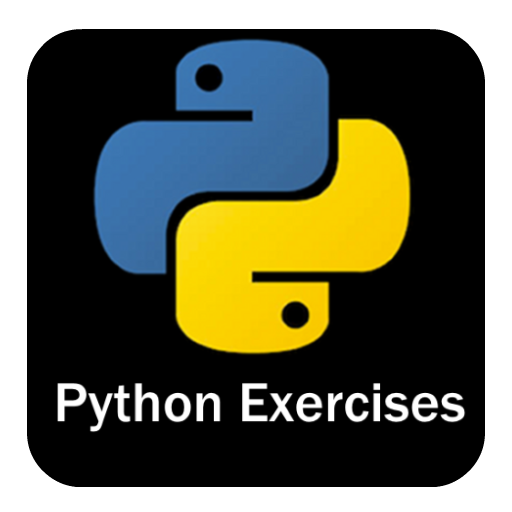# Dhananjay Kulkarni# Python Basics

## Part 2 : Python for Scripting

In the first part of our "Python for Scripting" series, we introduced Python, its use cases, and features, and even ran a simple "Hello World" script. In this second installment, we're going to dive deeper into Python and explore its fundamental building blocks. By the end of this blog post, you'll have a strong grasp of Python's basic syntax, data types, and control structures.

## Variables and Data Types

In Python, variables are used to store data. Unlike some other languages, you don't need to declare a variable's data type explicitly; Python infers it based on the value assigned to the variable. Let's look at some common data types:

### Integers and Floats

Integers are whole numbers, and floats are numbers with a decimal point.

Example:

``````# Integer
x = 5

# Float (floating-point number)
y = 3.14
``````

### Strings

Strings are used to represent text or characters.

Example:

``````message = "Hello, Python!"
``````

### Booleans

Booleans represent binary values - `True` or `False`.

Example:

``````is_python_fun = True
``````

Comments in Python start with the `#` symbol. They are ignored by the Python interpreter and are used to add explanations or notes to your code.

Example:

``````# This is a comment
``````

### Basic Operators

Python supports various operators for performing operations on data:

#### Arithmetic Operators

Example:

``````addition = 5 + 3  # 8
subtraction = 10 - 2  # 8
multiplication = 4 * 2  # 8
division = 8 / 4  # 2.0 (Note: Division always results in a float)
``````

#### Comparison Operators

Example:

``````equals = (5 == 5)  # True
not_equals = (5 != 3)  # True
greater_than = (7 > 3)  # True
less_than = (2 < 4)  # True
``````

#### Logical Operators

Example:

``````and_operator = (True and False)  # False
or_operator = (True or False)  # True
not_operator = (not True)  # False
``````

## Input and Output

Python provides functions for getting input from the user and displaying output. Here's a simple example:

Example:

``````name = input("What's your name? ")
print("Hello, " + name + "!")
``````

Expected Output:

``````What's your name? Dhananjay
Hello, Dhananjay!
``````

## Conditional Statements

Conditional statements allow you to make decisions in your code. The most common one is the `if` statement:

Example:

``````age = 18
if age >= 18:
print("You can vote!")
else:
print("You cannot vote yet.")
``````

Expected Output:

``````You can vote!
``````

## ExerciseNow that you've learned the basics, let's put your knowledge to the test with some exercises:

Exercise 1: Write a Python script that calculates the area of a rectangle. Prompt the user to enter the length and width as input.

Exercise 2: Create a program that checks whether a given number is even or odd and prints an appropriate message.

Exercise 3: Write a Python script that converts degrees Celsius to Fahrenheit. Prompt the user for the temperature in Celsius and display the result in Fahrenheit.

Exercise 4: Implement a simple calculator that can perform addition, subtraction, multiplication, and division. Prompt the user to enter two numbers and an operator (+, -, *, /).

Solution to Previous Exercise (Part 1):

In Part 1, I gave you the following exercise:

Write a Python script that asks the user for their name and then greets them with a personalized message. For example, if the user enters "Dhananjay," the script should print "Hello, Dhananjay!"

Here's a solution to that exercise:

``````# Get the user's name
name = input("What's your name? ")

# Greet the user
print("Hello, " + name + "!")
``````

Expected Output:

``````What's your name? Dhananjay
Hello, Dhananjay!
``````

For each exercise, provide a detailed solution with code and expected output in the comments section below. In the next blog post, we'll delve into Python data structures like lists and dictionaries. Stay tuned for more Python scripting goodness!

That's it for Part 2 of our Python for Scripting series. I hope you found this introduction to Python basics helpful. In the next installment, we'll explore Python data structures, so make sure to follow along. Happy coding!